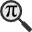## General

Display information for equation id:math.219374.43 on revision:219374

* Page found: Euler's formula (eq math.219374.43)

(force rerendering)

Cannot find the equation data in the database. Fetching from revision text.

Occurrences on the following pages:

Hash: b8a74bd4a57bf1d1068d3cb240498581

TeX (original user input):

i e ^{ix}


TeX (checked):

ie^{ix}


### LaTeXML (experimental; uses MathML) rendering

MathML (1.681 KB / 488 B) :$ie^{{ix}}$
<math xmlns="http://www.w3.org/1998/Math/MathML" id="p1.1.m1.1" class="ltx_Math" alttext="{\displaystyle ie^{{ix}}}" display="inline">
<semantics id="p1.1.m1.1a">
<mrow id="p1.1.m1.1.4" xref="p1.1.m1.1.4.cmml">
<mi id="p1.1.m1.1.1" xref="p1.1.m1.1.1.cmml">i</mi>
<mo id="p1.1.m1.1.4.1" xref="p1.1.m1.1.4.1.cmml">⁢</mo>
<msup id="p1.1.m1.1.4.2" xref="p1.1.m1.1.4.2.cmml">
<mi id="p1.1.m1.1.2" xref="p1.1.m1.1.2.cmml">e</mi>
<mrow id="p1.1.m1.1.3.1" xref="p1.1.m1.1.3.1.cmml">
<mi id="p1.1.m1.1.3.1.1" xref="p1.1.m1.1.3.1.1.cmml">i</mi>
<mo id="p1.1.m1.1.3.1.3" xref="p1.1.m1.1.3.1.3.cmml">⁢</mo>
<mi id="p1.1.m1.1.3.1.2" xref="p1.1.m1.1.3.1.2.cmml">x</mi>
</mrow>
</msup>
</mrow>
<annotation-xml encoding="MathML-Content" id="p1.1.m1.1b">
<apply id="p1.1.m1.1.4.cmml" xref="p1.1.m1.1.4">
<times id="p1.1.m1.1.4.1.cmml" xref="p1.1.m1.1.4.1"/>
<ci id="p1.1.m1.1.1.cmml" xref="p1.1.m1.1.1">𝑖</ci>
<apply id="p1.1.m1.1.4.2.cmml" xref="p1.1.m1.1.4.2">
<csymbol cd="ambiguous" id="p1.1.m1.1.4.2.1.cmml" xref="p1.1.m1.1.4.2">superscript</csymbol>
<ci id="p1.1.m1.1.2.cmml" xref="p1.1.m1.1.2">𝑒</ci>
<apply id="p1.1.m1.1.3.1.cmml" xref="p1.1.m1.1.3.1">
<times id="p1.1.m1.1.3.1.3.cmml" xref="p1.1.m1.1.3.1.3"/>
<ci id="p1.1.m1.1.3.1.1.cmml" xref="p1.1.m1.1.3.1.1">𝑖</ci>
<ci id="p1.1.m1.1.3.1.2.cmml" xref="p1.1.m1.1.3.1.2">𝑥</ci>
</apply>
</apply>
</apply>
</annotation-xml>
<annotation encoding="application/x-tex" id="p1.1.m1.1c">{\displaystyle ie^{{ix}}}</annotation>
</semantics>
[/itex]


SVG (2.96 KB / 1.316 KB) :

### MathML with SVG or PNG fallback (recommended for modern browsers and accessibility tools) rendering

SVG image empty. Force Re-Rendering

SVG (0 B / 8 B) :

PNG (0 B / 8 B) :

$ie^{ix}$## Translations to Computer Algebra Systems

### Translation to Maple

In Maple: i*(e)^(i*x)

I: You use a typical letter for a constant [the imaginary unit == the principal square root of -1].

We keep it like it is! But you should know that Maple uses I for this constant.

If you want to translate it as a constant, use the corresponding DLMF macro \iunit

e: the mathematical constant e == Napier's constant == 2.71828182845... was translated to: e

exp(1): You use a typical letter for a constant [the mathematical constant e == Napier's constant == 2.71828182845...].

We keep it like it is! But you should know that Maple uses exp(1) for this constant.

If you want to translate it as a constant, use the corresponding DLMF macro \expe

i: the imaginary unit == the principal square root of -1 was translated to: i

### Translation to Mathematica

In Mathematica: i*(e)^(i*x)

E: You use a typical letter for a constant [the mathematical constant e == Napier's constant == 2.71828182845...].

We keep it like it is! But you should know that Mathematica uses E for this constant.

If you want to translate it as a constant, use the corresponding DLMF macro \expe

I: You use a typical letter for a constant [the imaginary unit == the principal square root of -1].

We keep it like it is! But you should know that Mathematica uses I for this constant.

If you want to translate it as a constant, use the corresponding DLMF macro \iunit

e: the mathematical constant e == Napier's constant == 2.71828182845... was translated to: e

i: the imaginary unit == the principal square root of -1 was translated to: i

## Similar pages

Calculated based on the variables occurring on the entire Euler's formula page

## Identifiers

• $i$• $e$• $i$• $x$### MathML observations

0results

0results

no statistics present please run the maintenance script ExtractFeatures.php

0 results

0 results